# 6 Results

View
Selected filters:
• Hypotenuse
Remix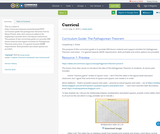Conditional Remix & Share Permitted
CC BY-NC
Rating

This is a remix of https://www.oercommons.org/authoring/29037-curriculum-guide-the-pythagorean-theorem/view by Shana Friend, with a few resources added at the beginning to provide more conceptual connections.

The purpose of this curriculum guide is to provide OER lesson material and support activities for Pythagorean Theorem instruction. It is geared towards GED® requirements. Both printable and online options are provided.

Subject:
Mathematics
Material Type:
Activity/Lab
Assessment
Lesson
Teaching/Learning Strategy
Author:
Susan Jones
08/08/2019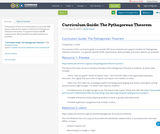Conditional Remix & Share Permitted
CC BY-NC
Rating

The purpose of this curriculum guide is to provide OER lesson material and support activities for Pythagorean Theorem instruction. It is geared towards GED® requirements. Both printable and online options are provided.

Subject:
Mathematics
Material Type:
Activity/Lab
Assessment
Lesson
Teaching/Learning Strategy
Author:
Shana Friend
05/30/2018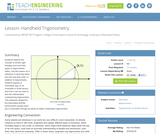Educational Use
Rating

Students explore the concept of similar right triangles and how they apply to trigonometric ratios. Use this lesson as a refresher of what trig ratios are and how they work. In addition to trigonometry, students explore a clinometer app on an Android® or iOS® device and how it can be used to test the mathematics underpinning trigonometry. This prepares student for the associated activity, during which groups each put a clinometer through its paces to better understand trigonometry.

Subject:
Engineering
Mathematics
Trigonometry
Material Type:
Lesson Plan
Provider:
TeachEngineering
Provider Set:
TeachEngineering
Author:
Scott Burns
09/18/2014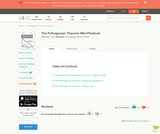Conditional Remix & Share Permitted
CC BY-NC
Rating

This Flexbook is community contributed through ck12.org. It covers three lessons on the Pythagorean Theorem. 1) Introduction and Determining if the Triangle is a Right Triangle, 2) Finding the Hypotenuse, and 3) Finding a leg. It includes step by step instructions, application problems, and answers (at the end of each lesson). Ck12.org material is downloadable, editable, and accessible offline and online.

Subject:
Mathematics
Algebra
Geometry
Material Type:
Lecture Notes
Lesson
Provider:
CK-12 Foundation
Provider Set:
CK-12 FlexBook
Author:
Shana Friend
05/25/2018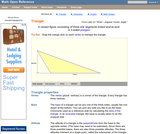Rating

An interactive applet and associated web page that introduce the concept of a triangle. The applet shows a triangle where the user can drag the vertices to reshape it. As it is being dragged a base and altitude are shown continuously changing. Demonstrates that the altitude may require the base to be extended. The text on the page lists the properties of a triangle and lists the various triangle types, with links to a definition of each. Applet can be enlarged to full screen size for use with a classroom projector. This resource is a component of the Math Open Reference Interactive Geometry textbook project at http://www.mathopenref.com.

Subject:
Geometry
Material Type:
Simulation
Provider:
Math Open Reference
Author:
John Page
02/16/2011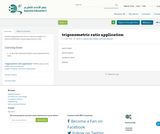Unrestricted Use
CC BY
Rating

student applying about how to find the height of a school building by using trigonometric ratio

Material Type:
Activity/Lab
Author:
Ayman Abu Delleh and Luai Alomari